# Seaborn - Linear Relationships Tutorial¶

Most of the time, we use datasets that contain multiple quantitative variables, and the goal of an analysis is often to relate those variables to each other. This can be done through the regression lines.

While building the regression models, we often check for multicollinearity, where we had to see the correlation between all the combinations of continuous variables and will take necessary action to remove multicollinearity if it exists. In such cases, the following techniques help.

#### Functions to Draw Linear Regression Models¶

There are two main functions in Seaborn to visualize a linear relationship determined through regression. These functions are regplot() and lmplot().

Example Plotting the regplot and then lmplot with the same data in this example

In :
import pandas as pd
import seaborn as sb
from matplotlib import pyplot as plt
sb.regplot(x = "total_bill", y = "tip", data = df)
sb.lmplot(x = "total_bill", y = "tip", data = df)
plt.show()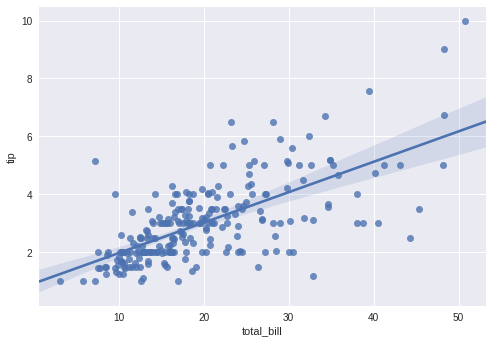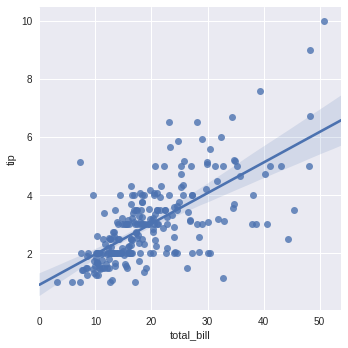You can see the difference in the size between two plots.

We can also fit a linear regression when one of the variables takes discrete values

In :
import pandas as pd
import seaborn as sb
from matplotlib import pyplot as plt
sb.lmplot(x = "size", y = "tip", data = df)
plt.show()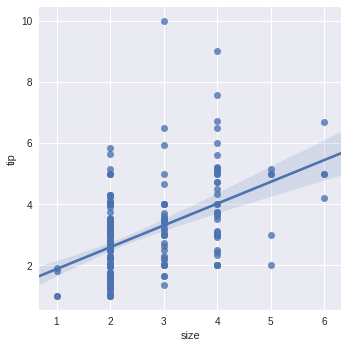### Fitting Different Kinds of Models¶

The simple linear regression model used above is very simple to fit, but in most of cases, the data is non-linear and the above methods cannot generalize the regression line.

Let us use Anscombe’s dataset with the regression plots −

In this case, the data is a good fit for the linear regression model with less variance.

Let us see another example where the data takes high deviation which shows the line of best fit is not good.

In :
import pandas as pd
import seaborn as sb
from matplotlib import pyplot as plt
sb.lmplot(x = "x", y = "y", data = df.query("dataset == 'II'"))
plt.show()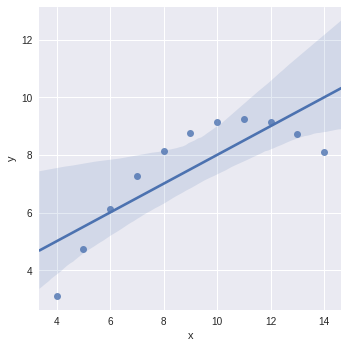The plot shows the high deviation of data points from the regression line. Such non-linear, higher-order can be visualized using the lmplot() and regplot().These can fit a polynomial regression model to explore simple kinds of nonlinear trends in the dataset.

In :
import pandas as pd
import seaborn as sb
from matplotlib import pyplot as plt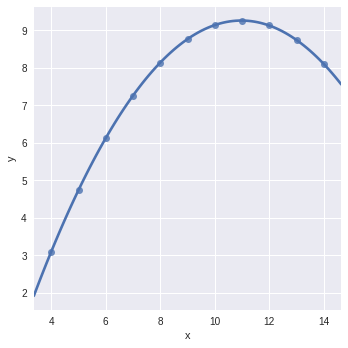Sunny Solanki